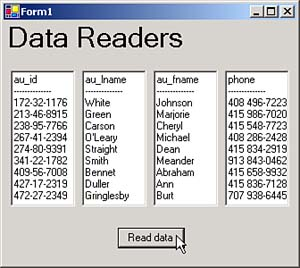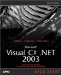Data readers let you read data from a data source field by field in a low-level way, and you use them when speed is an issue. You can create data readers only in codethere are no data reader objects in the toolbox. We'll take a look at data readers here in the ch10_04 example, which you can see at work in Figure 10.5. When the user clicks the Read Data button in this example, a data reader reads the data in the authors table field by field and we display that data in the text boxes you see in the figure.

##### Figure 10.5. The ch10_04 example.There are four types of data readers OleDbDataReader , SqlDataReader , OdbcDataReader , and OracleDataReader and we'll start this topic by taking a look at them in overview.

#### Working with the OleDbDataReader Class

The OleDbDataReader class creates a data reader for use with an OLE DB data provider. You can find the significant public properties of OleDbDataReader objects in Table 10.25, and their significant methods in Table 10.26.

##### Table 10.25. Significant Public Properties of OleDbDataReader Objects

PROPERTY

PURPOSE

FieldCount

Returns the number of columns in a row.

IsClosed

Returns True if a data reader is closed.

##### Table 10.26. Significant Public Methods of OleDbDataReader Objects

METHOD

PURPOSE

Close

GetBoolean

Returns a field's data as a Boolean.

GetByte

Returns a field's data as a byte.

GetBytes

GetChar

Returns a field's data as a character.

GetChars

Reads a stream of characters .

GetDateTime

Returns a field's data as a DateTime object.

GetDecimal

Returns a field's data as a Decimal object.

GetDouble

Returns a field's data as a double-precision floating-point number.

GetFieldType

Returns the data type of a field.

GetFloat

Returns a field's data as a single-precision floating-point number.

GetGuid

Returns a field's data as a globally unique identifier (GUID).

GetInt16

Returns a field's data as a 16-bit signed integer.

GetInt32

Returns a field's data as a 32-bit signed integer.

GetInt64

Returns a field's data as a 64-bit signed integer.

GetName

Returns the name of the given column.

GetOrdinal

Returns the column ordinal position.

GetSchemaTable

Returns an XML schema for a table.

GetString

Returns a field's data as a string.

GetValue

Returns the value of the column in its original format.

GetValues

Returns all the attribute columns in the current row.

IsDBNull

Returns True if a column contains non-existent (or missing) values.

Moves to the next record and reads that record.

#### Working with the SqlDataReader Class

The SqlDataReader class creates a data reader for use with the SQL Server; the SqlDataReader class has the same significant public properties and methods as the OleDbDataReader class, with the additional significant methods you see in Table 10.27.

METHOD

PURPOSE

GetSqlBinary

Returns a field's data as a SqlBinary .

GetSqlByte

Returns a field's data as a SqlByte .

GetSqlDateTime

Returns a field's data as a SqlDateTime .

GetSqlDecimal

Returns a field's data as a SqlDecimal .

GetSqlDouble

Returns a field's data as a SqlDouble .

GetSqlGuid

Returns a field's data as a SqlGuid .

GetSqlInt16

Returns a field's data as a SqlInt16 .

GetSqlInt32

Returns a field's data as a SqlInt32 .

GetSqlInt64

Returns a field's data as a SqlInt64 .

GetSqlMoney

Returns a field's data as a SqlMoney .

GetSqlSingle

Returns a field's data as a SqlSingle .

GetSqlString

Returns a field's data as a SqlString .

GetSqlValue

Returns an object of SqlDbType Variant .

GetSqlValues

Returns all the attribute columns in the current row.

#### Working with the OdbcDataReader Class

The OdbcDataReader class lets you create a data reader for use with an ODBC data provider. The OdbcDataReader class has the same significant public properties and methods as the OleDbDataReader class.

#### Working with the OracleDataReader Class

The OracleDataReader class lets you create a data reader for use with the Oracle data provider. The OracleDataReader class has the same significant public properties and methods as the OleDbDataReader class, with some additional significant methods, as you see in Table 10.28.

METHOD

PURPOSE

GetOracleBFile

Returns a field's data as an OracleBFile object.

GetOracleBinary

Returns a field's data as an OracleBinary object.

GetOracleDateTime

Returns a field's data as an OracleDateTime object.

GetOracleMonthSpan

Returns a field's data as an OracleMonthSpan object.

GetOracleNumber

Returns a field's data as an OracleNumber object.

GetOracleString

Returns a field's data as an OracleString object.

GetOracleTimeSpan

Returns a field's data as an OracleTimeSpan object.

GetOracleValue

Returns the value of a field in Oracle format.

#### Creating Data Readers in Code

You create data readers with the ExecuteReader method of a command object. In this example, we'll connect to the authors table using the OLE DB protocol. Here's how you create a new OLE DB data reader:

` `
` private void button1_Click(object sender, System.EventArgs e) {  string connection1String = "Provider=SQLOLEDB;" +   "Data Source=;User ID=sa;Initial Catalog=pubs;";   OleDbConnection connection1 = new OleDbConnection(connection1String);   OleDbCommand command1 = new OleDbCommand("select * from authors",   connection1);   connection1.Open();   OleDbDataReader reader1 =   command1.ExecuteReader(CommandBehavior.CloseConnection);  .     .     . `

This data reader gives us access to the authors table. If we want to reproduce the kind of field-by-field display we saw in the previous example, ch10_04, we'll need to start by getting the name of each column in the authors table. Unfortunately, data readers are very simple data objects, designed to return data from the fields in a table, one after the next. To get the names of the columns in a table takes a little more work, but it can be done. You do that by using the data reader's GetSchemaTable method to get the XML schema for the table, which lets you retrieve the name of each column this way:

` `
` private void button1_Click(object sender, System.EventArgs e) {   string connection1String = "Provider=SQLOLEDB;" +     "Data Source=;User ID=sa;Initial Catalog=pubs;";   OleDbConnection connection1 = new OleDbConnection(connection1String);     .     .     .  DataTable schemaTable = reader1.GetSchemaTable();   textBox1.Text += schemaTable.Rows.ToString() + "\r\n";   textBox2.Text += schemaTable.Rows.ToString() + "\r\n";   textBox3.Text += schemaTable.Rows.ToString() + "\r\n";   textBox4.Text += schemaTable.Rows.ToString() + "\r\n";   textBox1.Text += "--------------" + "\r\n";   textBox2.Text += "--------------" + "\r\n";   textBox3.Text += "--------------" + "\r\n";   textBox4.Text += "--------------" + "\r\n";  .     .     . `

Data readers return the data from field after field in your data source. You use methods like GetBoolean , GetString , and GetDouble to read the actual data from a data reader, which means you must know the data type of the field you're fetching data from. You can determine that type using the XML schema for the table so you know the data-reading method to use. Here's what that looks like in the ch10_04 example, where we're checking for both string and boolean values:

` `
` private void button1_Click(object sender, System.EventArgs e) {   string connection1String = "Provider=SQLOLEDB;" +     "Data Source=;User ID=sa;Initial Catalog=pubs;";   OleDbConnection connection1 = new OleDbConnection(connection1String);     .     .     .  while (reader1.Read())   {   if (schemaTable.Rows.ToString() == "System.String") {   textBox1.Text += reader1.GetString(0) + "\r\n";   }   if (schemaTable.Rows.ToString() == "System.Boolean") {   textBox1.Text += reader1.GetBoolean(0).ToString() + "\r\n";   }   if (schemaTable.Rows.ToString() == "System.String") {   textBox2.Text += reader1.GetString(1) + "\r\n";   }   if (schemaTable.Rows.ToString() == "System.Boolean") {   textBox2.Text += reader1.GetBoolean(1).ToString() + "\r\n";   }   if (schemaTable.Rows.ToString() == "System.String") {   textBox3.Text += reader1.GetString(2) + "\r\n";   }   if (schemaTable.Rows.ToString() == "System.Boolean") {   textBox3.Text += reader1.GetBoolean(2).ToString() + "\r\n";   }   if (schemaTable.Rows.ToString() == "System.String")   {   textBox4.Text += reader1.GetString(3) + "\r\n";   }   if (schemaTable.Rows.ToString() == "System.Boolean") {   textBox4.Text += reader1.GetBoolean(3).ToString() + "\r\n";   }   }  reader1.Close();   connection1.Close(); } `

You can see the results of this code in Figure 10.5, where you see the first four fields of the records in the authors table.Microsoft Visual C#.NET 2003 Kick Start
ISBN: 0672325470
EAN: 2147483647
Year: 2002
Pages: 181

Similar book on Amazon